CALCULATION

Force

A force is a push or pull that can cause an object with mass to accelerate.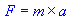force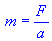mass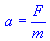acceleratio

The SI unit of Force is Newton (N)
The SI unitof mass is Kilogram (Kg)
The SI unit of accelerationis the meter per second squared (m/s2)

Work

Work is the amount of energy transferred by a force.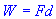Work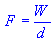Force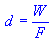distance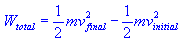Total work

Where
 W = Work Wtotal = total work F = force D = distance M = mass vinitial = initial velocity vfinal = final velocity

The SI unit of workis the Joule (J), which is defined as the work done by a force of one newton acting over a distance of one metre.
The SI unit of Force is Newton (N)
The SI unitof mass is Kilogram (Kg)
The SI unit of distanceis the meter (m)
The SI unit of velocityis the meter per second (m/s)

POWER

Power is the rate at which work is done.
The SI units for mechanical and electrical Power are the Watt (W), and the Watt (electrical) (We) respectively.

Power is the rate of doing work, or at which energy is converted. It can be thought of as - "amount of energy used to perform work, or converted from one form to another, in a specified period of time, namely, in a second"

Power and work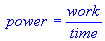power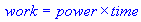work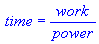time

Power and displacement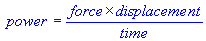Power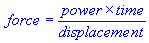Force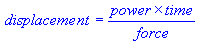Displacement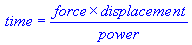Time

Power and velocity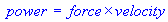Power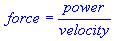Force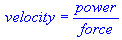Velocity

Kinetic Energy

Kinetic Energy is defined as the work needed to accelerate a body of a given mass from rest to its current velocity.
The kinetic energy of an object is the energy which it possesses due to its motion.It is defined as the work needed to accelerate a body of a given mass from rest to its stated velocity. Having gained this energy during its acceleration, the body maintains this kinetic energy unless its speed changes. The same amount of work is done by the body in decelerating from its current speed to a state of rest.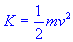Kinetic energy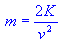mass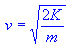velocity

Where

Ke  = Kinetic Energy
M  = mass
v  = Velocity

Potential energy

Potential energy is the stored energy of position possessed by an object.
In physics, potential energy is the energy of an object or a system due to the position of the body or the arrangement of the particles of the system.The SI unit for measuring work and energy is the Joule (symbol J).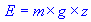Potential energy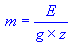Mass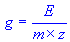Acceleration of gravity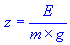Height

Centrifugal Acceleration

Acceleration directed toward the center of a circular path. This is called as Centripetal or Centrifugal acceleration.

# Centrifugal acceleration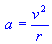Centrifugalacceleration (meter per second squared)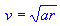Velocity (meter per second)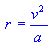Radius (meter)

Centrifugal force

Centrifugal forceis the apparent outward force that draws a rotating body away from the center of rotation. It is caused by the inertia of the body as the body's path is continually redirected. In Newtonian mechanics, the term centrifugal force is used to refer to one of two distinct concepts: an inertial force observed in a non-inertial reference frame, and a reaction force corresponding to a centripetal force.

Centripetal Force:
If  m = Mass,  r = Circular Radius, v = Velocity.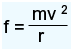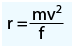Velocity: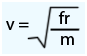Circular Velocity

The velocity at which a body must move in order to maintain an orbit at the outer edge of the earth's atmosphere. This velocity is called as Circular Velocity.

Circular Velocity:

Circular velocity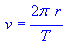circular velocity (meter per second)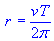Radius (in meter)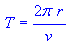Period (in second)

Velocity

If
v0 = initial velocity
v  = final velocity
vave = average velocity
a  = acceleration
t  = time
?x = distance or displacement

Velocity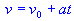velocity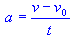acceleration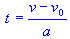time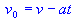initial velocity

Average Velocity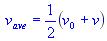average velocity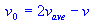initial velocity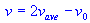velocity

Displacement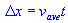distance or displacement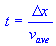time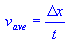average velocity

Kinetic frictionKinetic frictionKinetic friction coefficientNormal force

Static frictionStatic frictionStatic friction coefficientNormal force

Newton Law of Gravity

Newton Law of Gravity states that Every particle attracts every other particle with a force that is proportional to the product of their masses and inversely proportional to the distance between them.

Newton's law of gravityGravitational force exerted between two objectsMass of object 1Mass of object 2Distance between the objects

Kepler's Third Law states that The squares of the orbital periods of planets are directly proportional to the cubes of the semi-major axis of the orbits.Satellite orbit periodSatellite mean orbital radiusPlanet mass

Escape Velocity is the minimum velocity needed to escape the gravitational field of a planet or other body. It is also called as Critical or Escape Speed.Escape or critical speedPlanet massPlanet radius